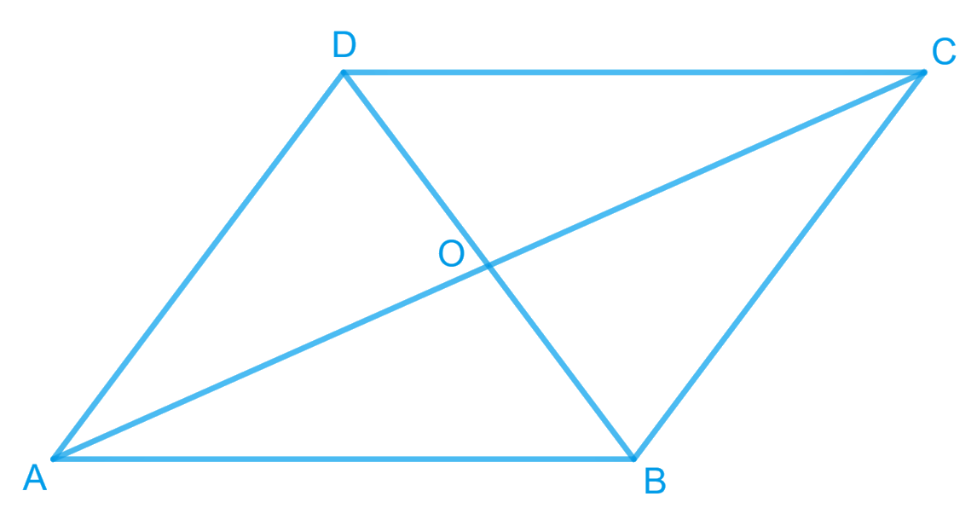# Ex.9.3 Q3 Areas of Parallelograms and Triangles Solution - NCERT Maths Class 9

Go back to  'Ex.9.3'

## Question

Show that the diagonals of a parallelogram divide it into four triangles of equal area.

Video Solution
Areas Of Parallelograms And Triangles
Ex 9.3 | Question 3

## Text Solution

What is known?

Parallelogram and its diagonals.

What is unknown?

How we can show that the diagonals of a parallelogram divide it into four triangles of equal area.

Reasoning:

We know that diagonals of a parallelogram bisect each other it means we will get mid- point for both the diagonals through which medians will be formed. Also, median divides the triangle in two triangles of equal areas. By using these observations, we can show the required result.

Steps:We know that the diagonals of a parallelogram bisect each other.

Therefore, $$O$$ is the mid-point of $$AC$$ and $$BD$$.

$$BO$$ is the median in $$\Delta ABC$$. Therefore, it will divide it into two triangles of equal areas.

Therefore

$\text{ ar }\!\!(\Delta AOB)\!\!= \!\!\text{ar } (\Delta BOC) \ldots (1)$

In $$\Delta BCD$$, $$CO$$ is the median.

Therefore

$\text{ ar }\!(\Delta BOC)\!\!=\!\!\text{ar } (\Delta COD) \ldots(2)$

Similarly,

Therefore

$\text{ ar }\!(\Delta COD)\!=\!\text{ar }\!(\Delta AOD) \ldots(3)$

From Equations ($$1$$), ($$2$$), and ($$3$$), we obtain

\begin{align}\text{Area } (\Delta AOB) &= \text{Area } (\Delta BOC)\\& =\text{Area } (\Delta COD)\\& = \text{Area } (\Delta AOD)\end{align}

Therefore, it is evident that the diagonals of a parallelogram divide it into four triangles of equal area.

Video Solution
Areas Of Parallelograms And Triangles
Ex 9.3 | Question 3